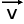# Point Vector Length node

Left Toolbar >Math Nodes >Point Vector Length

The Point Vector Length node allows you to calculate the vector length.

## Procedure

### To calculate a point vector length in the node graph:

1. On the left toolbar choose Point Nodes> Point Vector Length2. Drag the Point Vector Length icon into the Node Graph.3. Connect the point Nodes to the vector input.

4. Connect the Output: result .

## Inputs

vector A POINT type of value.

## Outputs

result

A POINT type of value.(x,y)

## Attributes PanelVector

This attribute is exposed as input by default. Right-click the Node to hide this attribute as input and set the point values manually.

Math Nodes

Main Page